Maths-
General
Easy

Question

# A and B can finish a work in 20 days. A alone can do 1/5th of the work in 12 days. In how many days can B alone do it?Hint:

## The correct answer is: 30 days.

### Let’s say that the total work can be represented as W and let’s say the amount of work that A can do in one day is WA and the amount of work that B can do in one day is WB.Step 1 of 3:It is given that A alone can do 1/5th of the work in 12 days.So, Work done by A in one day(WA) =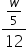=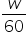…….(1)Step 2 of 3:It is given that A and B can finish a work in 20 days. So we can writeWA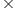20 + WB20 = WUsing equation (1)+ WB  =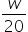WB  =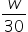Step 3 of 3:Now, the time taken by B completeamount of work = 1 daySo, time taken by B to complete W amount of work =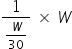= 30 daysFinal Answer: Hence, the time that will B to complete the whole work is 30 days.#### With Turito Foundation.#### Get an Expert Advice From Turito.